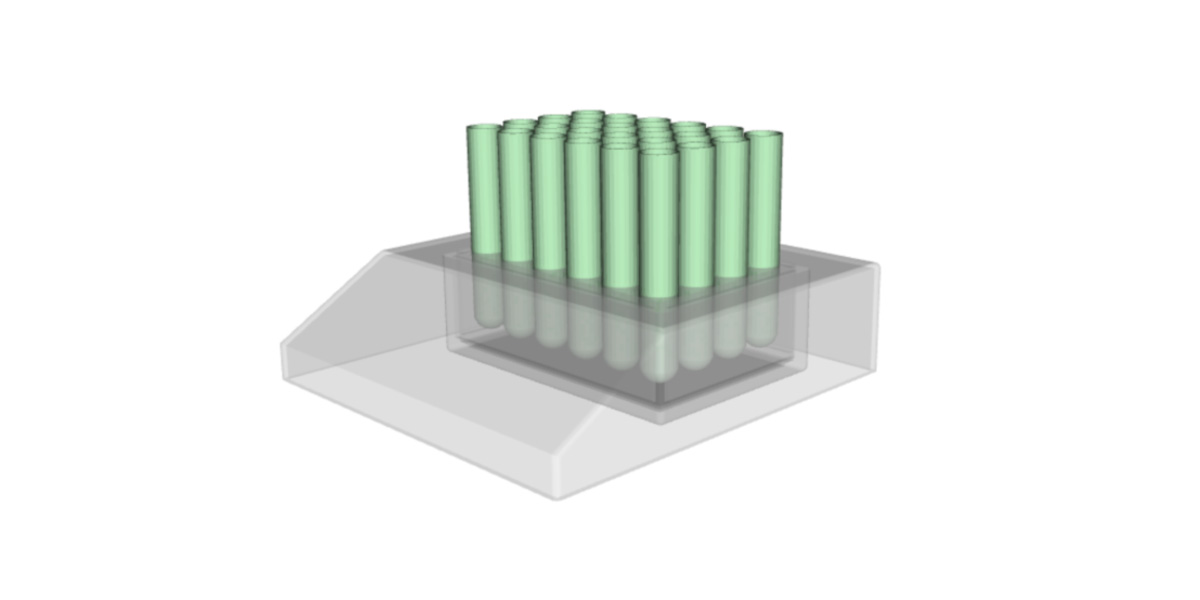Blog

News

## Electric Heating Element Performance Targets | Diabaitx

February 24, 2023by
Niels VerdijckWith the winter coming to a close (in the northern hemisphere), this article might seem oddly timed to discuss electric heating elements and their design process. However, there are still plenty of applications left that require an efficient electric heating element. For example, the heating element used to heat up battery cells to their optimal operating temperatures as quickly as possible during the start-up of a battery electric vehicle. Or the well-known tire blankets in different racing categories where the main goal is mainly to keep the temperatures as close as possible to the desired operating conditions.

In this article, we’ll explore the most important performance targets when designing electric heating elements.

### Different application groups

There are two main groups of electric heating elements:

1. The first group of heaters operates in a transient region where the users require a certain heat-up time to be limited. An example application is the battery cell heaters, as discussed in the introduction.
2. The second group of heaters operates in the steady state regime, where its users want to keep their products as close as possible to a desired target temperature for a prolonged time. An example application of this group is, for example, a test tube heater.

### Transient heater elements

The transient heater elements are only used briefly to heat an application in a short period of time. The main performance value is thus the heat-up time expressed in seconds (in SI units).

In transient analysis, it is well-known that any transient system will become steady state after five times the time constant . This transforms thus into the following equation:

$t_{\mathrm{heatup}}=5 \times \tau_\mathrm{c}=\frac{5}{Q/(\rho c_\mathrm{p} V(T_{\mathrm{initial}}-T_{\mathrm{target}})}$

Where $$\tau_\mathrm{c}$$ is the time constant, $$Q$$ is the heat flux supplied by the heater, $$\rho$$ and $$c_\mathrm{p}$$ are the density and heat capacity of the material to heat up, $$V$$ its volume and $$T_{\mathrm{initial}}$$ and $$T_{\mathrm{target}}$$ are the initial and target temperatures.

From this equation, it is clear that adding more heat will result in a slower heat-up. But this is a rather inefficient solution (especially given the current energy challenges). A better solution would be one where the material is cleverly placed. Regions with high thermal inertia should have a dense wire pattern compared to areas with low thermal inertia.

Steady-state heaters operate very much in a different manners. Instead of heating a component as quickly as possible, these elements combat the heat losses to the environment. As with any application that operates at a higher temperature than its environment, the thermodynamic laws state that the system will try to balance itself by transferring heat from the hot object to the colder one. The regions with high thermal transmittance to the environment should thus have locally more wire material placed in the regions that don’t have a high thermal transmittance.

The main objective of these heater elements is to keep the application as close as possible to a desired operating temperature. The further away from this operating temperature, the worse the design. This translates into the following penalization scheme:

$J=\frac{1}{V}\int_{V}(T-T_{\mathrm{operating}})^2 dV$

Where $$T$$ is the actual temperature distribution of the application and $$V$$, its volume; and $$T_{\mathrm{operating}}$$ is the desired operation temperature.

### Conclusion

The targets used to evaluate electric heater elements very much depends on the type of heating element one needs to design. A short head up time is desired for transient heating elements, where the main counterforce is the thermal inertia of the application. For steady state heating elements, temperature uniformity is the most important design target. For steady state heating elements, a non-uniform heat loss to the environment requires the most thought-out design.

Coldstream has the expertise to handle these diverse design targets for electric heater elements, ensuring optimal performance and efficiency for any application. If you want to access how ColdStream can help with your workflow, please contact us here.

References: